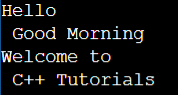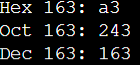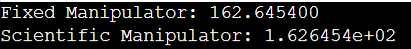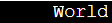# Manipulators in C++

## Manipulators in C++ with Examples:

In this article, I am going to discuss Manipulators in C++ with Examples. Please read our previous article where we discussed Text and Binary Files in C++ with Examples.

##### What are Manipulators in C++?

Manipulators are helping functions in C++ that are used to modify the input/output stream. What it means, it will not modify the value of a variable, it will only modify the streams or formatting streams using the insertion (<<) and extraction (>>) operators.

1. Manipulators are special functions that can be included in the I/O statement to alter the format parameters of a stream.
2. Manipulators are operators that are used to format the data display.
3. To access manipulators, the file iomanip should be included in the program.

Manipulators are used for enhancing streams or formatting streams. For writing the data, we can adopt some formats. For example, a common manipulator that we used is the endl that is used for the endline. Instead of endl, we can also say that cout << “\n”; This will also print a new line. So, endl is a manipulator which is used for formatting stream. So, it is useful for formatting output streams.

##### Example to understand \n Manipulator in C++:
```#include <iostream>
#include <iomanip>
using namespace std;
int main()
{
cout << "Hello \n Good Morning\n";
cout << "Welcome to \n C++ Tutorials";
return 0;
}
```
###### Output:##### Integer Manipulators in C++:

Now let us see what are other manipulators available. There are some manipulators available for data types like integer and float. For integer type data, we have manipulators,

1. hex – it will display the data in hexadecimal.
2. oct – it will display data in the octal form.
3. dec – to display data in decimal form.

For example, if we say cout << hex << 163;

The output of the above statement will be A3. The hexadecimal form of 163 is A3. So, we can mention the manipulator then the output will be in that form. So, all the integers will be in the hexadecimal form that is written after the manipulator. And if you want to change the number system then you have to mention decimal, octal anything that you want. For a better understanding, please have a look at the below example.

```#include <iostream>
#include <iomanip>
using namespace std;
int main()
{
cout << "Hex 163: " << hex << 163 <<"\n";
cout << "Oct 163: " << oct << 163 <<"\n";
cout << "Dec 163: " << dec << 163 <<"\n";
return 0;
}
```
###### Output:##### Float Manipulators in C++:

Now, similarly, for floating points, we have manipulators,

1. Fixed: It will show in the fixed floating-point number. For example cout << fixed << 162.6454; Then the same number will be displayed.
2. scientific: It will display the number in scientific form or exponent form. For example cout << fixed << 162.6454; Then the scientific form or exponent form of this number i.e. 1.626454e+02 will be displayed.

For a better understanding, please have a look at the below example.

```#include <iostream>
#include <iomanip>
using namespace std;
int main()
{
cout << "Fixed Manipulator: " << fixed << 162.6454 <<endl;
cout << "Scientific Manipulator: " <<scientific << 162.6454 <<"\n";
return 0;
}
```
###### Output:There are other manipulators also available. They are as follows:

setw – It will set some amount of space for displaying the data. For example,

cout << setw(10) << “World”;

This “World” will be displayed in 10 spaces. Though the number of the alphabet is only 5, it will be shown in 10 places. For a better understanding, please have a look at the below example.

```#include <iostream>
#include <iomanip>
using namespace std;
int main()
{
cout << setw(10) << "World";
return 0;
}
```
###### Output: